# Solving Equations of Motion for Systems of Particles with MATLAB (Part -2) Civil Engineering (CE) Notes | EduRev

## Civil Engineering (CE) : Solving Equations of Motion for Systems of Particles with MATLAB (Part -2) Civil Engineering (CE) Notes | EduRev

The document Solving Equations of Motion for Systems of Particles with MATLAB (Part -2) Civil Engineering (CE) Notes | EduRev is a part of the Civil Engineering (CE) Course Introduction to Dynamics and Vibrations- Notes, Videos, MCQs.
All you need of Civil Engineering (CE) at this link: Civil Engineering (CE)

Example 3: Silly FE exam problem

This example shows how polar coordinates can be used to analyze motion.

The rod shown in the picture rotates at constant angular speed in the horizontal plane.  The interface between block and rod has friction coefficient μ .  The rod pushes a block of mass m, which starts at r=0 with radial speed V.   Find an expression for r(t).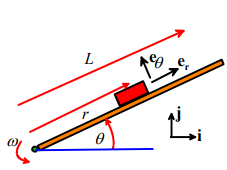1. Introduce variables to describe the motion – the polar coordinates r,θ work for this problem

2. Write down the position vector and differentiate to find acceleration – we don’t need to do this – we can just write down the standard result for polar coordinates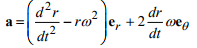3. Draw a free body diagram – shown in the figure – note that it is important to draw the friction force in the correct direction. The block will slide radially outwards, and friction opposes the slip.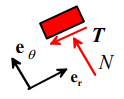4. Write down Newton’s law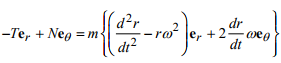5. Eliminate reactions

F=ma gives two equations for N and T.   A third one comes from the friction law T = μN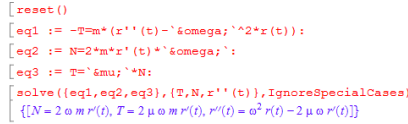The third solution can be rearranged into an equation of motion for r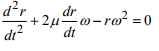6. Identify initial conditions Here, r=0 dr/dt=V at time t=0.

7. Solve the equation: If you’ve taken AM33 you will know how to solve this equation… But if not, or you are lazy, you can use MAPLE to solve it for you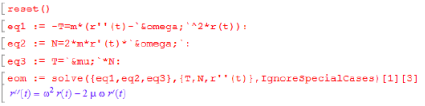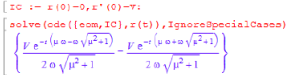This can be simplified slightly by hand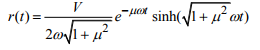Example 4: Motion of a pendulum (R-rated version)

A pendulum is a ubiquitous engineering system. You are, of course, familiar with how a pendulum can be used to measure time. But it’s used for a variety of other scientific applications. For example, Professor Crisco’s lab uses pendulum to measure properties of human joints, see Crisco Joseph J; Fujita Lindsey; Spenciner David B, ‘The dynamic flexion/extension properties of the lumbar spine in vitro using a novel pendulum system.’ Journal of biomechanics 2007;40(12):2767-73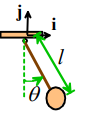In this example, we will work through the basic problem of deriving and solving the equations of motion for a pendulum, neglecting air resistance.

1. Introduce variables to describe motion: The angle θ(t) shown in the figure is a convenient variable.

2. Write down the position vector as a function of the variables We introduce a Cartesian coordinate system with origin at O, as shown in the picture.

Elementary geometry shows that r = l sinθ i-l cosθ j

Note that we have assumed that the cable remains straight – this will be true as long as the internal force in the cable is tensile.  If calculations predict that the internal force is compressive, this assumption is wrong.  But there is no way to check the assumption at this point so we simply proceed, and check the answer at the end

3. Differentiate the position vector to find the acceleration: The computer makes this painless.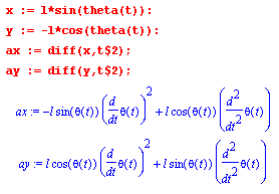4. The free body diagram is shown in the figure.   The force exerted by the cable on the particle is introduced as an unknown reaction force. The force vector is  F = -R sinθ i + (R cosθ - mg) j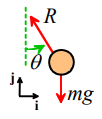5. Newton’s laws of motion can be expressed as F = ma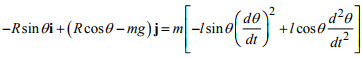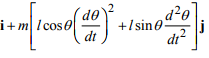Equating the i and j components gives two equations for the two unknowns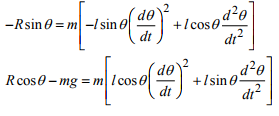6. Eliminate the reaction forces. – In this problem, it is helpful to eliminate the unknown reaction force R. You can do this on the computer if you like, but in this case it is simpler to do this by hand. You can simply multiply the first equation by cosθ and the second equation by sinθ and then add them. This yields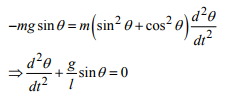7. Identify initial conditions. Some calculations are necessary to determine the initial conditions in this problem. We are given that θ = 0 at time t=0, and the horizontal velocity is V0 at time t=0, but to solve the equation of motion, we need the value of dθ / dt = 0 . We can find the relationship we need by differentiating the position vector to find the velocity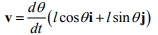Setting v = v0i and θ = 0 at t=0 shows that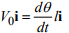so dθ / dt = V0 /l

8. Solve the equations of motion This equation of motion is too difficult for MAPLE but actually the solution does exist and is very well known – this is a classic problem in mathematical physics. With initial conditions θ = 0, dθ / dt = V0 / l   t = 0 the solution is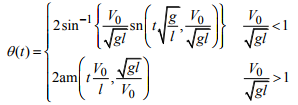The first solution describes swinging motion of the pendulum, while the second solution describes the motion that occurs if you push the pendulum so hard that it whirls around on the pivot. The equations may look scary, but you can simply use MAPLE to calculate and plot them.

1. In the first equation, sn(x,k) is a special function called the `sin amplitude.’ You can think of it as a sort of trig function for adults – in fact for k=0, sn(x,0) = sin(x)  and we recover the PG version. You can compute it in Mupad using jacobiSN (x,k)

2. Similarly, am(x,k) is a function called the `Amplitude.’ You can calculate it in Mupad using jacobiAM (x,k). In Mupad, the am function has range -π<am(x,k)<π , so the solution predicts that as the pendulum whirls around the pivot, the angle θ increases from 0 to 2π , then jumps to -2π , increases to 2π again, and so on.

You might have solved the pendulum problem already in an elementary physics course, and might remember a different solution. This is because you probably only derived an approximate solution, by assuming that the angle θ remains small. This occurs when the initial velocity satisfies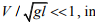which case the solution can be approximated by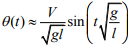3.3.3 Numerical solutions to equations of motion using MATLAB

In the preceding section, we were able to solve all our equations of motion exactly, and hence to find formulas that describe the motion of the system. This should give you a warm and fuzzy feeling – it appears that with very little work, you can predict everything about the motion of the system. You may even have visions of running a consulting business from your yacht in the Caribbean, with nothing more than your chef, your masseur (or masseuse) and a laptop with a copy of MAPLE.

Unfortunately real life is not so simple. Equations of motion for most engineering systems cannot be solved exactly. Even very simple problems, such as calculating the effects of air resistance on the trajectory of a particle, cannot be solved exactly.

For nearly all practical problems, the equations of motion need to be solved numerically, by using a computer to calculate values for the position, velocity and acceleration of the system as functions of time. Vast numbers of computer programs have been written for this purpose – some focus on very specialized applications, such as calculating orbits for spacecraft (STK); calculating motion of atoms in a material (LAMMPS); solving fluid flow problems (e.g. fluent, CFDRC); or analyzing deformation in solids (e.g. ABAQUS, ANSYS, NASTRAN, DYNA); others are more general purpose equation solving programs.

In this course we will use MATLAB, which is widely used in all engineering applications. You should complete the MATLAB tutorial before proceeding any further.

In the remainder of this section, we provide a number of examples that illustrate how MATLAB can be used to solve dynamics problems. Each example illustrates one or more important technique for setting up or solving equations of motion.

Example 1: Trajectory of a particle near the earth’s surface (with air resistance)

As a simple example we set up MATLAB to solve the particle trajectory problem discussed in the preceding section. We will extend the calculation to account for the effects of air resistance, however. We will assume that our projectile is spherical, with diameter D, and we will assume that there is no wind. You may find it helpful to review the discussion of aerodynamic drag forces in Section 2.1.7 before proceeding with this example.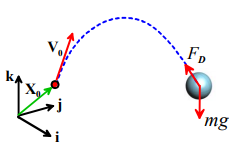1. Introduce variables to describe the motion: We can simply use the Cartesian coordinates of the particle (x(t), y(t), z(t))

2. Write down the position vector in terms of these variables: r = x(t)i + y(t) j + z(t)k

3. Differentiate the position vector with respect to time to find the acceleration. Simple calculus gives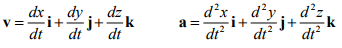4. Draw a free body diagram. The particle is now subjected to two forces, as shown in the picture. Gravity – as always we have Fg = -mgk.

Air resistance.

The magnitude of the air drag force is given by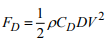, where

• p is the air density,
• CD is the drag coefficient,
• D is the projectile’s diameter, and
• V is the magnitude of the projectile’s velocity relative to the air. Since we assumed the air is stationary, V is simply the magnitude of the particle’s velocity, i.e.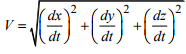The Direction of the air drag force is always opposite to the direction of motion of the projectile through the air. In this case the air is stationary, so the drag force is simply opposite to the direction of the particle’s velocity. Note that v /V is a unit vector parallel to the particle’s velocity. The drag force vector is therefore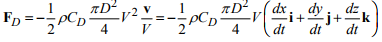The total force vector is therefore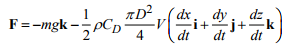5. Write down Newton’s laws of motion.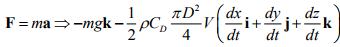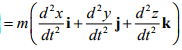It is helpful to simplify the equation by defining a specific drag coefficient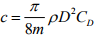, so that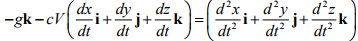The vector equation actually represents three separate differential equations of motion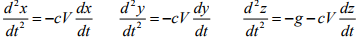6. Eliminate reactions – this is not needed in this example.

7. Identify initial conditions. The initial conditions were given in this problem - we have that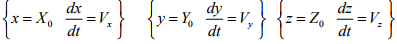8. Solve the equations of motion. We can’t use the magic ‘dsolve’ command in MAPLE to solve this equation – it has no known exact solution. So instead, we use MATLAB to generate a numerical solution.

This takes two steps. First, we must turn the equations of motion into a form that MATLAB can use. This means we must convert the equations into first-order vector valued differential equation of the general form dt/dt = f(t,y). Then, we must write a MATLAB script to integrate the equations of motion.

Converting the equations of motion: We can’t solve directly for (x,y,z), because these variables get differentiated more than once with respect to time. To fix this, we introduce the time derivatives of (x,y,z) as new unknown variables. In other words, we will solve for  (x, y, z, vx , vy , vz) , where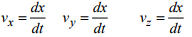These definitions are three new equations of motion relating our unknown variables. In addition, we can re-write our original equations of motion as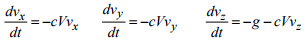So, expressed as a vector valued differential equation, our equations of motion are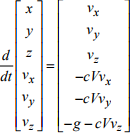MATLAB script. The procedure for solving these equations is discussed in the MATLAB tutorial. A basic MATLAB script is listed below.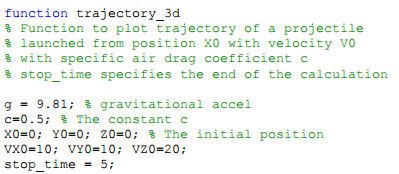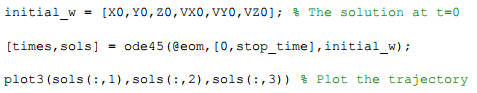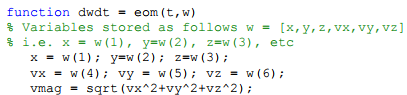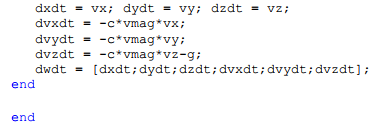This produces a plot that looks like this (the plot’s been edited to add the grid,etc)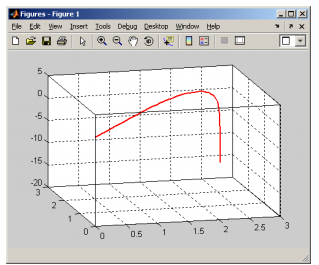Offer running on EduRev: Apply code STAYHOME200 to get INR 200 off on our premium plan EduRev Infinity!

## Introduction to Dynamics and Vibrations- Notes, Videos, MCQs

20 videos|53 docs

,

,

,

,

,

,

,

,

,

,

,

,

,

,

,

,

,

,

,

,

,

;